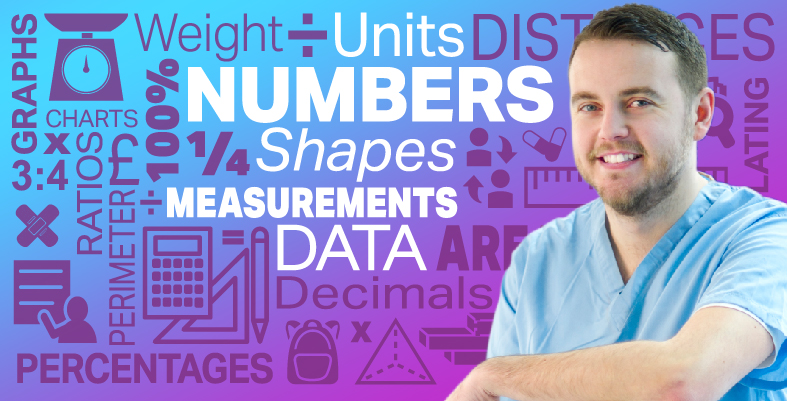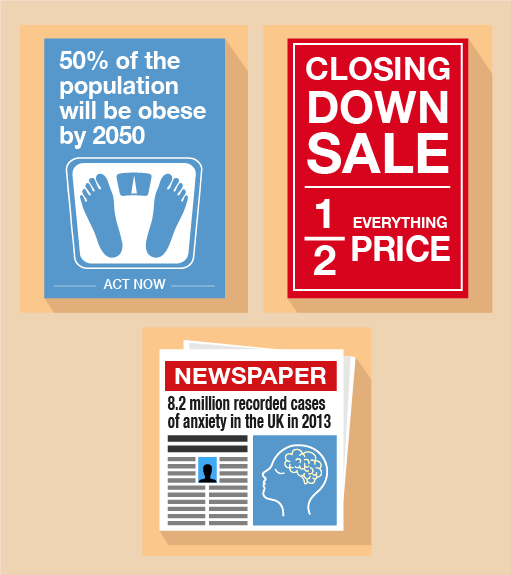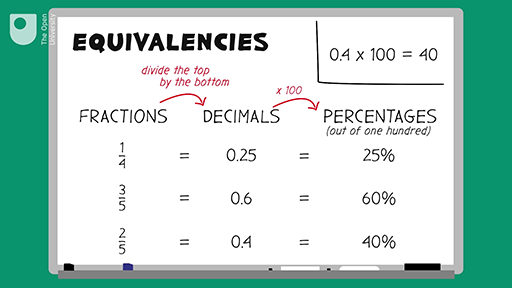Everyday maths for Health and Social Care and Education Support 1

Start this free course now. Just create an account and sign in. Enrol and complete the course for a free statement of participation or digital badge if available.

Free course

# 6 Equivalencies between fractions, decimals and percentagesFigure 25 Looking at equivalencies

Fractions, decimals and percentages are different ways of saying the same thing. It’s an important skill to learn about the relationships (or ‘equivalencies’) between fractions, decimals and percentages to make sure you are getting the better deal.Skip transcript

#### Transcript

In this video, you’re going to look at equivalent fractions, decimals and percentages. First, let’s look at turning fractions into decimals. You have the fraction one quarter. To turn this into a decimal, you need to divide the top of the fraction by the bottom. 1 divided by 4 equals 0.25. Therefore, 0.25 is the equivalent decimal.

Let’s have a look at another fraction, three fifths. (Remember, divide the top of the fraction by the bottom.) 3 divided by 5 equals 0.6. So 0.6 is the equivalent decimal.

Now let’s try one last fraction see if you can calculate it before the answer is revealed! Two fifths: divide the top by the bottom. 2 divided by 5 equals 0.4. So the decimal is 0.4.

Now let’s use the decimals that we’ve calculated to find the percentage equivalent. A percentage is out of 100. Remember, ‘cent’ means 100. So to turn a decimal into a percentage, you need to multiply the decimal by 100.

For example, 0.25 times 100 equals 25. This is 25%.

0.6 times 100 equals 60, 60%.

0.4 times 100 equals 40. This is 40%.

Now, looking at the table, you can see how fractions, decimals and percentages relate to each other. You just need to remember the basic rules of turning a fraction into a decimal: you divide the top by the bottom, then, once you have the decimal, you can convert to per cent by multiplying by 100, remembering that ‘cent’ means 100.

Thank you for watching. Now you have a go.

End transcript

Interactive feature not available in single page view (see it in standard view).

Here are some common equivalencies. Try to memorise them – you will come across them a lot in everyday situations:

10% = = 0.1

20% = = 0.2

25% = = 0.25

50% = = 0.5

75% = = 0.75

100% = 1 = 1.0

Look at the following example. If you can identify equivalences, they’ll make it easier to make simple calculations.

## Example: Mine’s a half

What is 50% of £200?

### Method

Since 50% is the same as , so:

50% of £200 = of £200 = £100

## Activity 17: Looking for equivalencies

1. What is 20% of £600?
2. If you walked 0.25 km each day, what fraction of a kilometre have you walked?
3. It is recommended that of a healthy meal should consist of fruit and vegetables. What is this as a percentage?
4. A medical supplier is holding a ‘10% off’ sale on hospital beds. How much would you pay for a new hospital bed worth £8,000 in the sale?
5. Approximately 20% of cases of long-term sickness absence at work are related to mental health conditions. Out of 3,000 cases of long-term sickness absence, work out how many are likely to be a result of a mental health condition.

1. 20% is the same as .

• 600 ÷ 5 = 120

So 20% of £600 is £120.

2. 0.25 is the same as . There are 1,000 m in 1 km.

• 1,000 ÷ 4 = 250

So walking 0.25 km is the same as m, simplified to , or of a kilometre.

3. is the same as 50%.

So 50% of a healthy meal should consist of fruit and vegetables.

4. 10% is the same as .

• 8,000 ÷ 10 = 800

So the bed has been reduced by £800 in the sale:

• £8,000 – £800 = £7,200

The bed will cost £7,200 in the sale.

5. 20% is the same as .

• 3,000 ÷ 5 = 600

Out of 3,000 cases of long-term sickness absence, 600 are likely to be a result of a mental health condition

Knowing the common equivalencies between fractions, decimals and percentages is important when trying to compare discounts when shopping or interpreting statistics related to health and social care.

## Summary

In this section you have learned about common equivalencies between fractions, decimals and percentages.

FSM_SSH_1